# From last time Position velocity and acceleration velocity

• Slides: 31
Download presentationFrom last time • Position, velocity, and acceleration – velocity = time rate of change of position – acceleration = time rate of change of velocity – Particularly useful concepts when • velocity is constant (undisturbed motion) • acceleration is constant (free falling object) HW#1: Due HW#2: Chapter 3: Conceptual: # 2, 26, 30, 40 Chapter 3: Problems: # 4, 6, 17 Physics 107, Fall 2006 1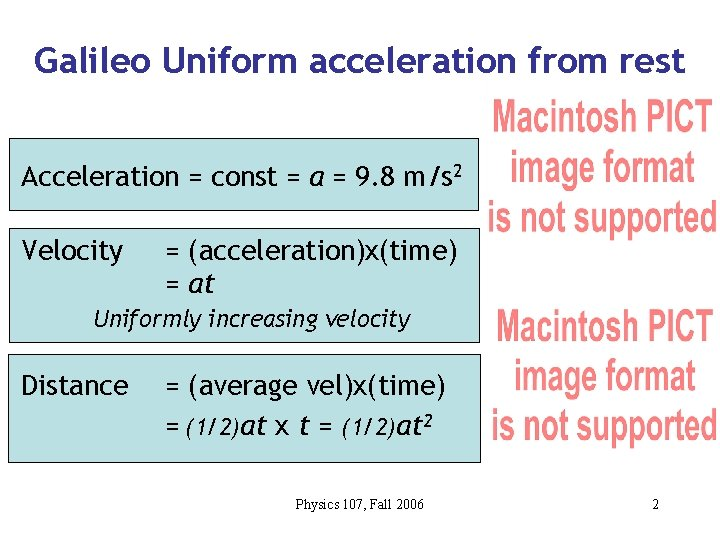Galileo Uniform acceleration from rest Acceleration = const = a = 9. 8 m/s 2 Velocity = (acceleration)x(time) = at Uniformly increasing velocity Distance = (average vel)x(time) = (1/2)at x t = (1/2)at 2 Physics 107, Fall 2006 2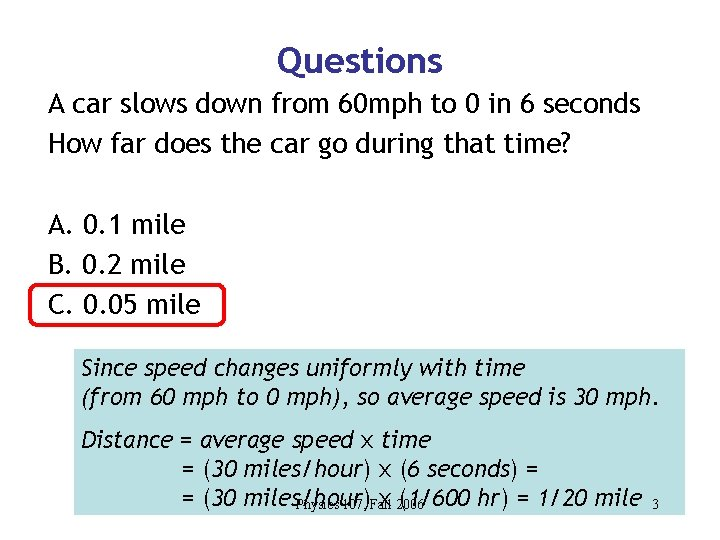Questions A car slows down from 60 mph to 0 in 6 seconds How far does the car go during that time? A. 0. 1 mile B. 0. 2 mile C. 0. 05 mile Since speed changes uniformly with time (from 60 mph to 0 mph), so average speed is 30 mph. Distance = average speed x time = (30 miles/hour) x (6 seconds) = = (30 miles/hour) x 2006 (1/600 hr) = 1/20 mile Physics 107, Fall 3Falling object: constant acceleration • Falling objects have constant acceleration. • This is called the acceleration of gravity 9. 8 m/s/s = 9. 8 m/s 2 • But why does gravity result in a constant acceleration? • Why is this acceleration independent of mass? Physics 107, Fall 2006 4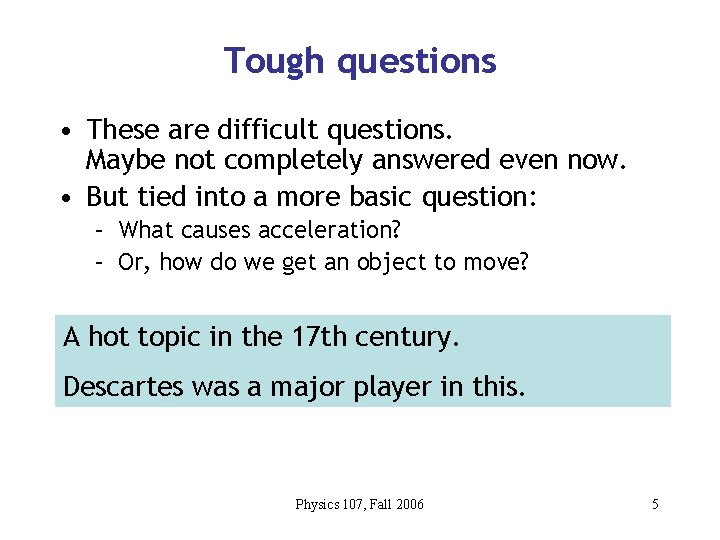Tough questions • These are difficult questions. Maybe not completely answered even now. • But tied into a more basic question: – What causes acceleration? – Or, how do we get an object to move? A hot topic in the 17 th century. Descartes was a major player in this. Physics 107, Fall 2006 5Descartes’ view… • Motion and rest are primitive states of a body without need of further explanation. • Bodies only change their state when acted upon by an external cause. This is similar our concept of inertia That a body, upon coming in contact with a stronger one, loses none of its motion; but that, upon coming in contact with a weaker one, it loses as much as it transfers to that weaker body Momentum and it’s conservation Physics 107, Fall 2006 6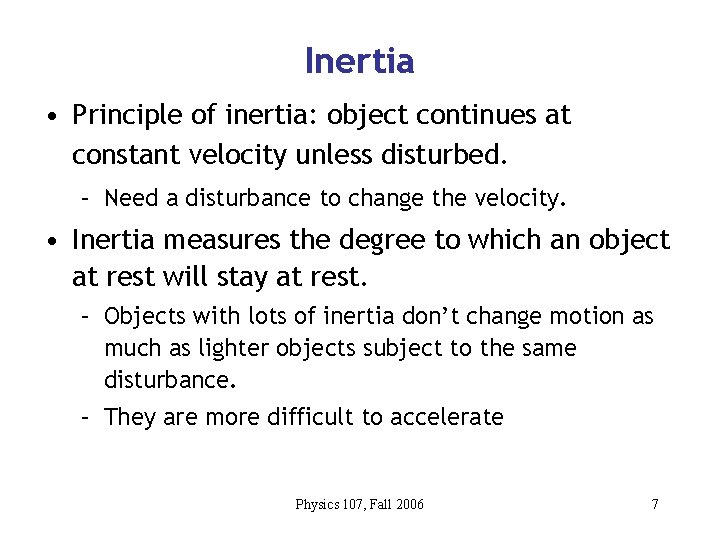Inertia • Principle of inertia: object continues at constant velocity unless disturbed. – Need a disturbance to change the velocity. • Inertia measures the degree to which an object at rest will stay at rest. – Objects with lots of inertia don’t change motion as much as lighter objects subject to the same disturbance. – They are more difficult to accelerate Physics 107, Fall 2006 7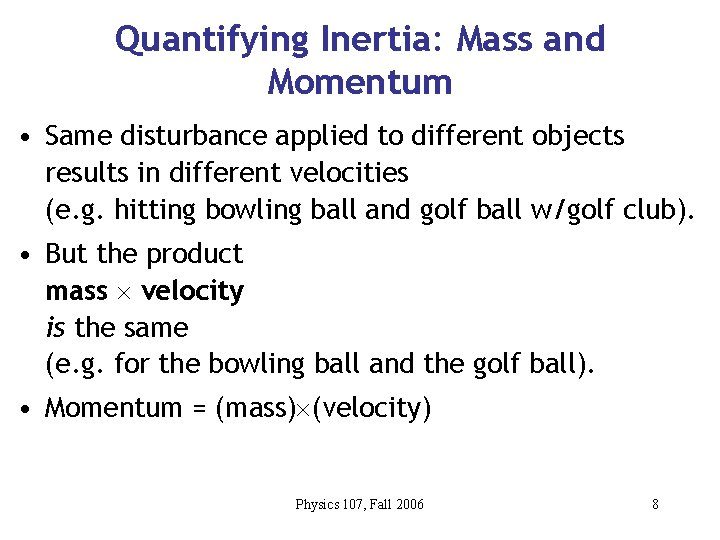Quantifying Inertia: Mass and Momentum • Same disturbance applied to different objects results in different velocities (e. g. hitting bowling ball and golf ball w/golf club). • But the product mass velocity is the same (e. g. for the bowling ball and the golf ball). • Momentum = (mass) (velocity) Physics 107, Fall 2006 8Momentum conservation • Can easily describe interactions of objects. • The total momentum (sum of momenta of each object) of the system is always the same. • We say that momentum is conserved. – Between the golf ball and the golf club • Momentum can be transferred from one object to the other, but it does not disappear. Physics 107, Fall 2006 9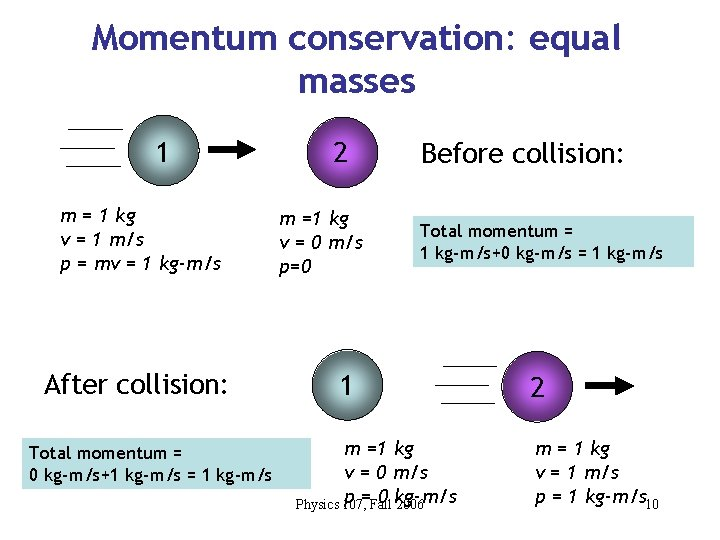Momentum conservation: equal masses 1 m = 1 kg v = 1 m/s p = mv = 1 kg-m/s After collision: Total momentum = 0 kg-m/s+1 kg-m/s = 1 kg-m/s 2 m =1 kg v = 0 m/s p=0 Before collision: Total momentum = 1 kg-m/s+0 kg-m/s = 1 kg-m/s 1 m =1 kg v = 0 m/s p =Fall 0 kg-m/s Physics 107, 2006 2 m = 1 kg v = 1 m/s p = 1 kg-m/s 10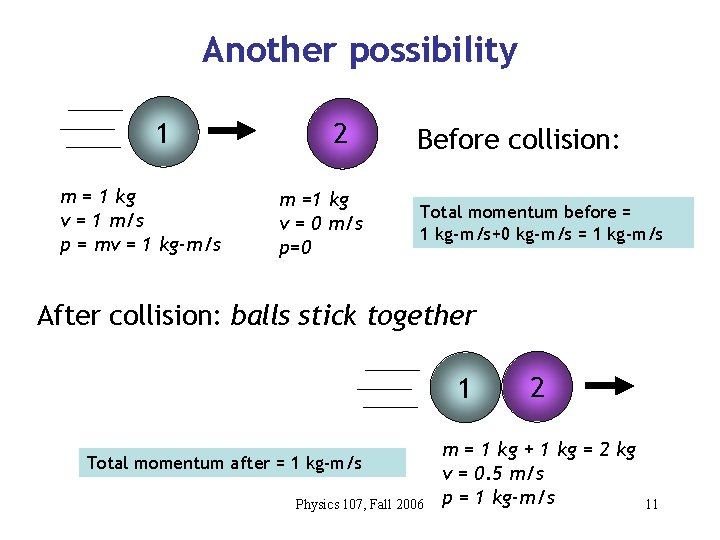Another possibility 1 m = 1 kg v = 1 m/s p = mv = 1 kg-m/s 2 m =1 kg v = 0 m/s p=0 Before collision: Total momentum before = 1 kg-m/s+0 kg-m/s = 1 kg-m/s After collision: balls stick together 1 Total momentum after = 1 kg-m/s Physics 107, Fall 2006 2 m = 1 kg + 1 kg = 2 kg v = 0. 5 m/s p = 1 kg-m/s 11Question Two ice skaters, Joe and Jane, are initially at rest. Joe is more massive than Jane. They push off of each other. Compared to Jane, Joe is moving A. faster B. slower C. the same. The momentum before they push off is zero, so it also is after they push off. The momenta must be equal and opposite. Since Joe is more massive, and Physics 107, Fall 2006 momentum = mass x velocity, his speed is slower. 12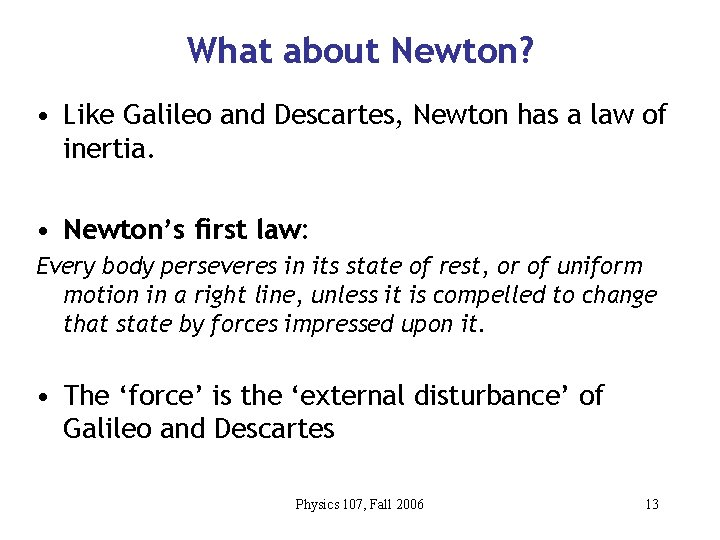What about Newton? • Like Galileo and Descartes, Newton has a law of inertia. • Newton’s first law: Every body perseveres in its state of rest, or of uniform motion in a right line, unless it is compelled to change that state by forces impressed upon it. • The ‘force’ is the ‘external disturbance’ of Galileo and Descartes Physics 107, Fall 2006 13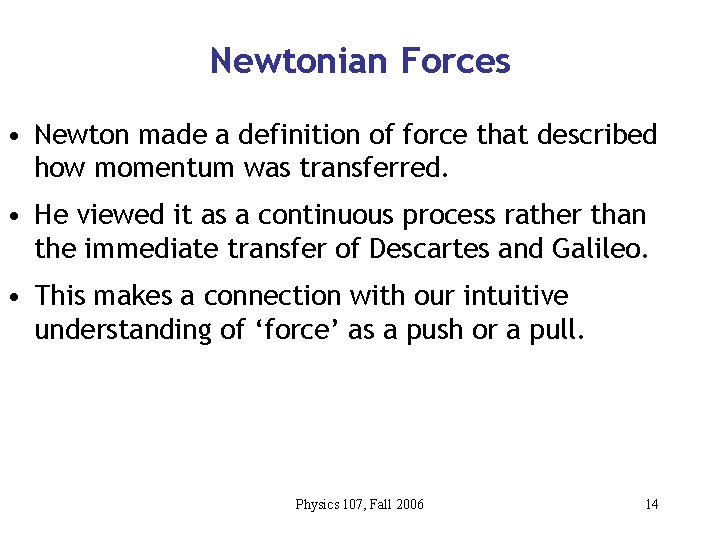Newtonian Forces • Newton made a definition of force that described how momentum was transferred. • He viewed it as a continuous process rather than the immediate transfer of Descartes and Galileo. • This makes a connection with our intuitive understanding of ‘force’ as a push or a pull. Physics 107, Fall 2006 14Newton’s second law • The change in motion is proportional to the motive force impressed; and is made in the direction of the right line in which that force is impressed. (Momentum change)=(Applied force) (time interval) Change in momentum = p Applied force = F Time interval = t Physics 107, Fall 2006 p = F t 15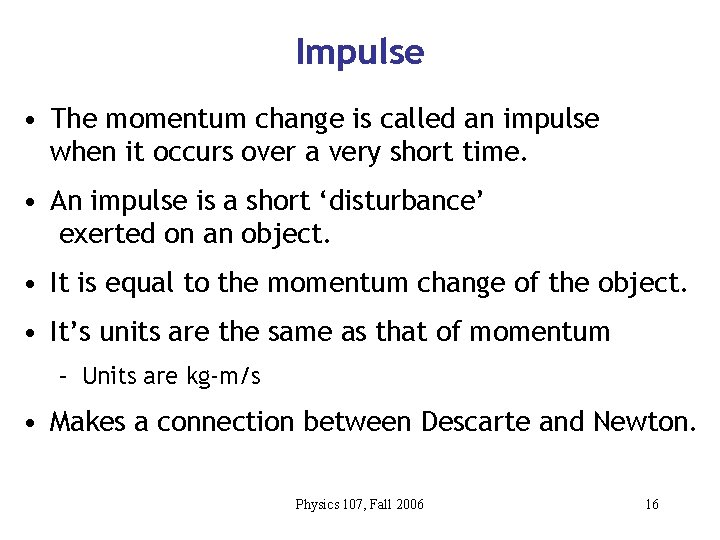Impulse • The momentum change is called an impulse when it occurs over a very short time. • An impulse is a short ‘disturbance’ exerted on an object. • It is equal to the momentum change of the object. • It’s units are the same as that of momentum – Units are kg-m/s • Makes a connection between Descarte and Newton. Physics 107, Fall 2006 16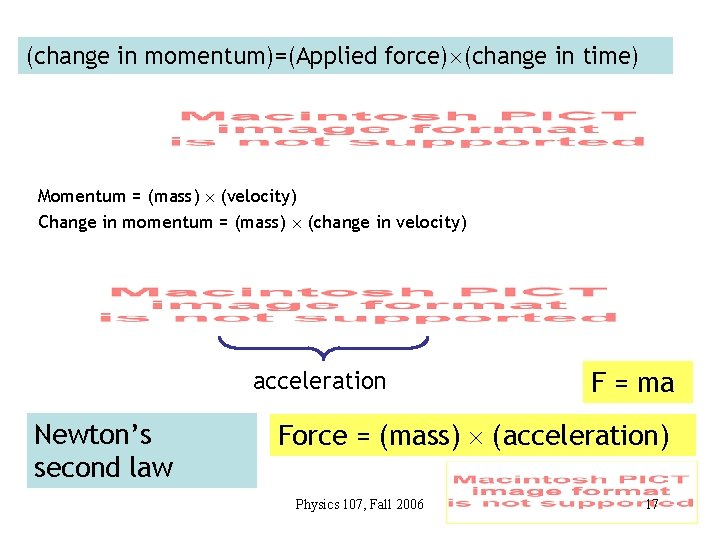(change in momentum)=(Applied force) (change in time) Momentum = (mass) (velocity) Change in momentum = (mass) (change in velocity) acceleration Newton’s second law F = ma Force = (mass) (acceleration) Physics 107, Fall 2006 17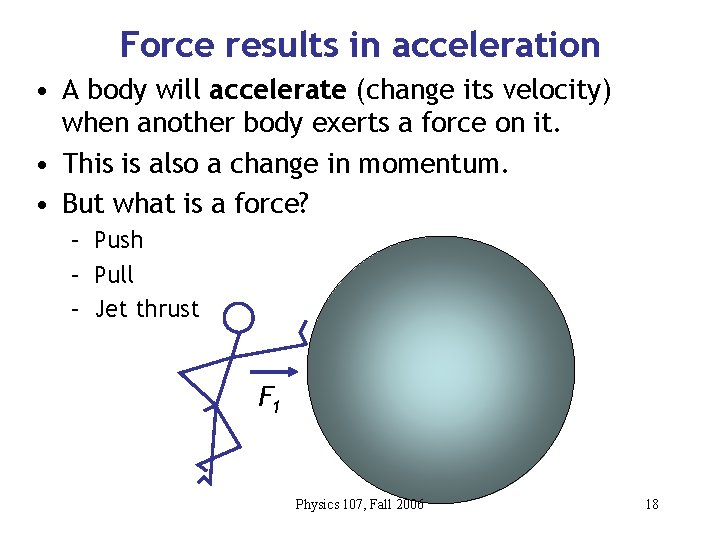Force results in acceleration • A body will accelerate (change its velocity) when another body exerts a force on it. • This is also a change in momentum. • But what is a force? – Push – Pull – Jet thrust F 1 Physics 107, Fall 2006 18More than one force… • Total force determines acceleration • If F 1 and F 2 balance, acceleration is zero. F 1 F 2 Physics 107, Fall 2006 19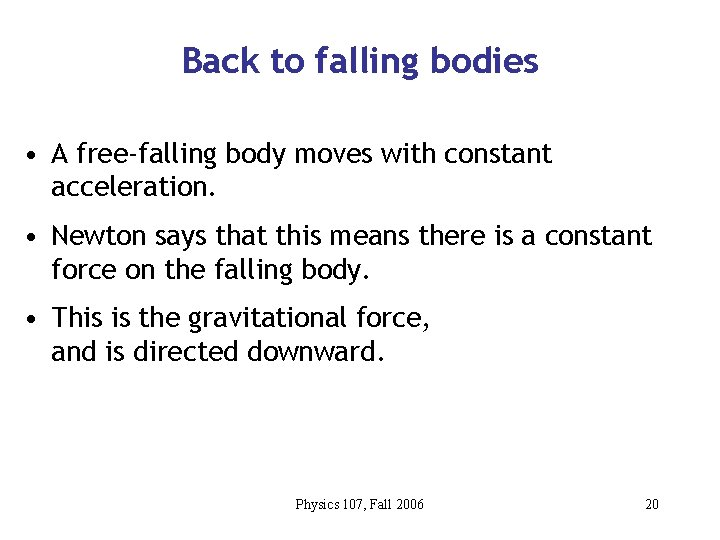Back to falling bodies • A free-falling body moves with constant acceleration. • Newton says that this means there is a constant force on the falling body. • This is the gravitational force, and is directed downward. Physics 107, Fall 2006 20Question When the vectron hovers near the ceiling, the propeller force compared to hovering near the floor is A. Greater. B. Less. C. The same. Gravity exerts a force downward. When the vectron hovers, its velocity is constant, so the acceleration is zero. This means the net force is zero. The propeller Physics 107, Fall 2006 force 21 force balances the gravitational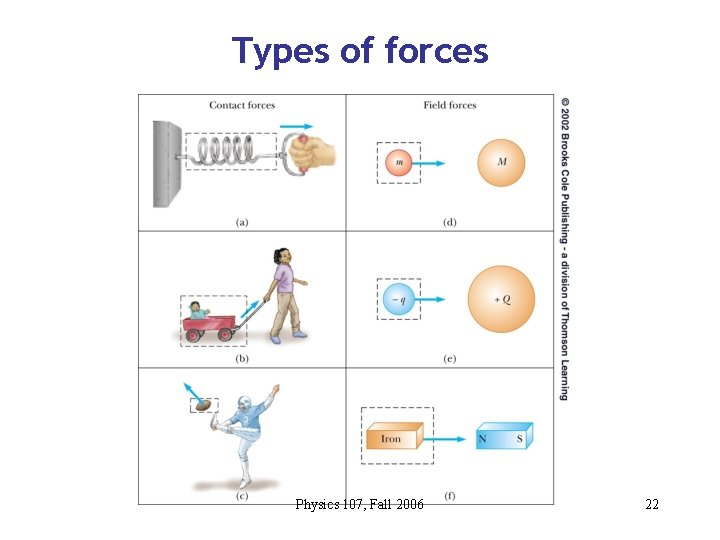Types of forces Physics 107, Fall 2006 22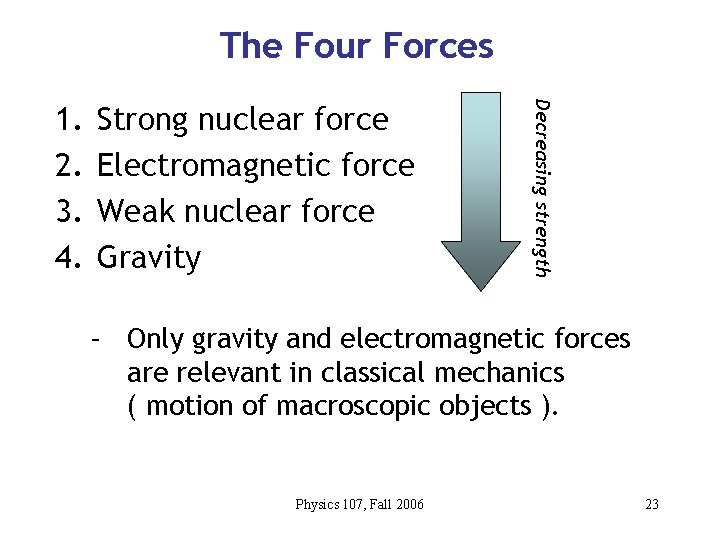The Four Forces Strong nuclear force Electromagnetic force Weak nuclear force Gravity Decreasing strength 1. 2. 3. 4. – Only gravity and electromagnetic forces are relevant in classical mechanics ( motion of macroscopic objects ). Physics 107, Fall 2006 23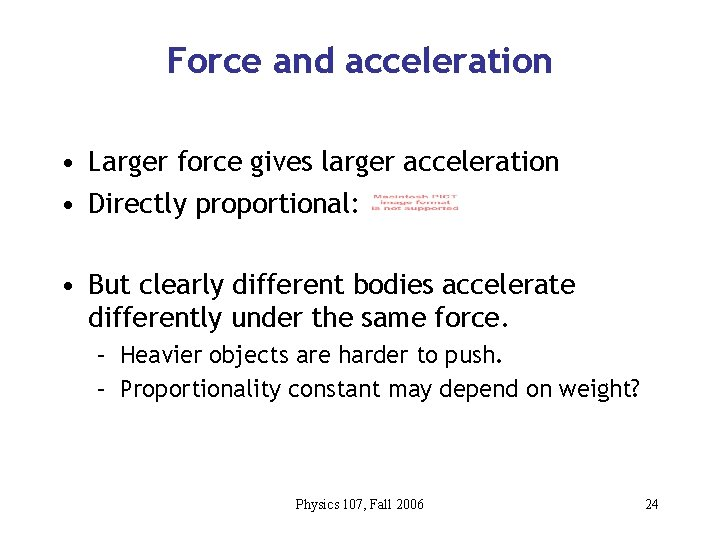Force and acceleration • Larger force gives larger acceleration • Directly proportional: • But clearly different bodies accelerate differently under the same force. – Heavier objects are harder to push. – Proportionality constant may depend on weight? Physics 107, Fall 2006 24Inertia again • But we already said that inertia characterizes a body’s tendency to retain its motion (I. e. to not change its velocity), We say a heavier object has more inertia. • But inertia and weight are different – A body in space is weightless, but it still resists a push Physics 107, Fall 2006 25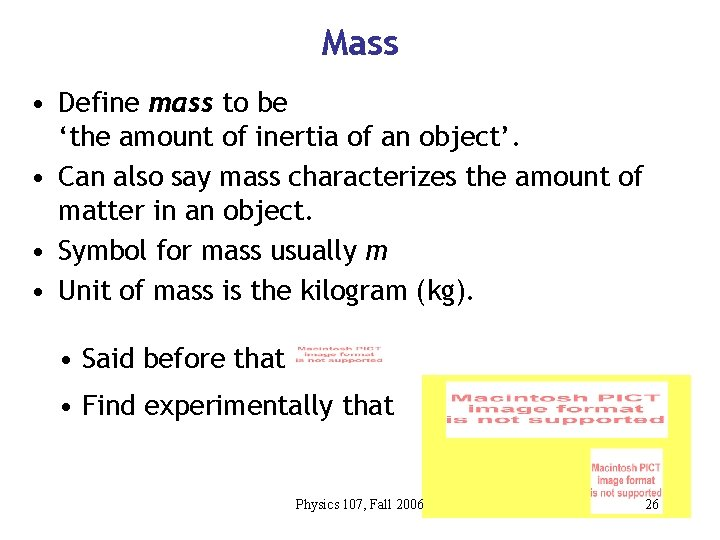Mass • Define mass to be ‘the amount of inertia of an object’. • Can also say mass characterizes the amount of matter in an object. • Symbol for mass usually m • Unit of mass is the kilogram (kg). • Said before that • Find experimentally that Physics 107, Fall 2006 26Force, weight, and mass • 1 Newton = force required to accelerate a 1 kg mass at 1 m/s 2. But then what is weight? —Weight is a force, measured in Newton’s —It is the net force of gravity on a body. —F=mg, g=F/m Physics 107, Fall 2006 27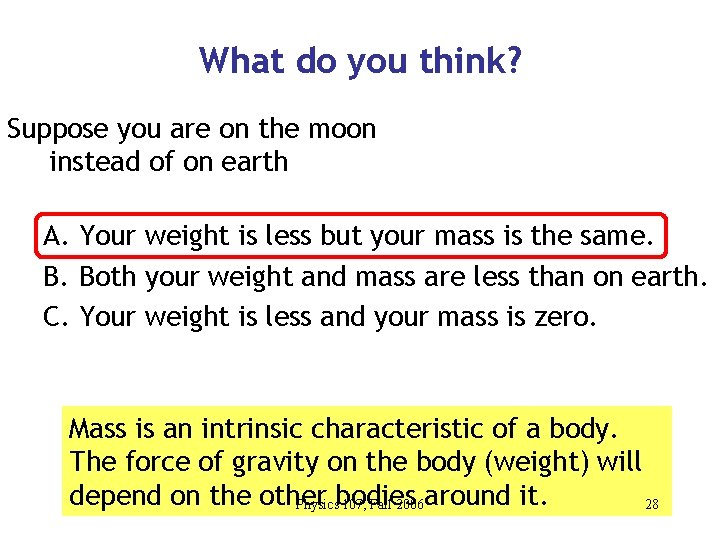What do you think? Suppose you are on the moon instead of on earth A. Your weight is less but your mass is the same. B. Both your weight and mass are less than on earth. C. Your weight is less and your mass is zero. Mass is an intrinsic characteristic of a body. The force of gravity on the body (weight) will depend on the other Physicsbodies 107, Fall 2006 around it. 28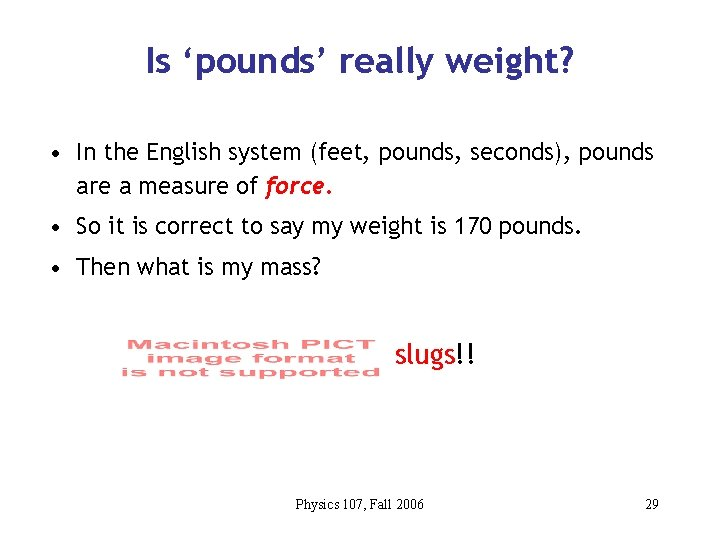Is ‘pounds’ really weight? • In the English system (feet, pounds, seconds), pounds are a measure of force. • So it is correct to say my weight is 170 pounds. • Then what is my mass? slugs!! Physics 107, Fall 2006 29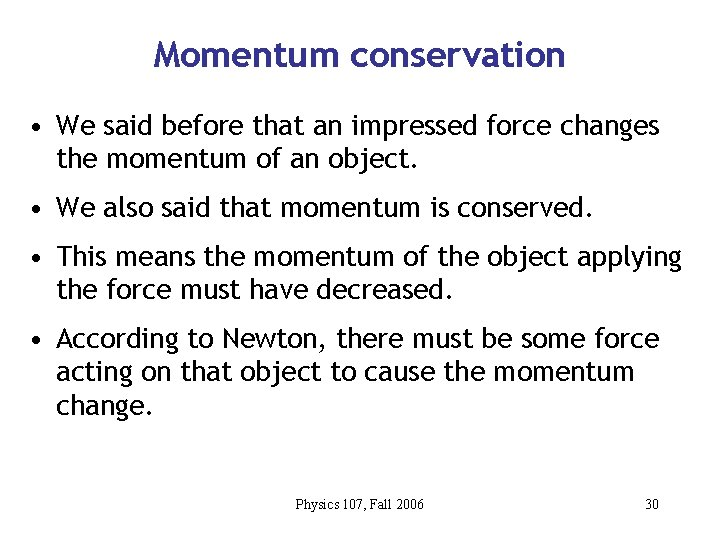Momentum conservation • We said before that an impressed force changes the momentum of an object. • We also said that momentum is conserved. • This means the momentum of the object applying the force must have decreased. • According to Newton, there must be some force acting on that object to cause the momentum change. Physics 107, Fall 2006 30Newton’s third law • This is the basis for Newton’s third law: To every action there is always opposed an equal reaction. This is momentum conservation in the language of forces. Physics 107, Fall 2006 31